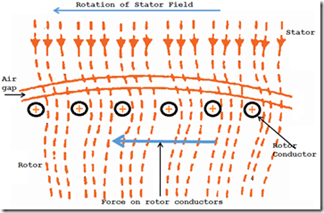# Principle Operation of Three Phase Induction MotorThe figure shows the portion of a three phase Induction motor and is principle explained below:
• The three phase stator winding gets energized when a three phase supply is given to the stator winding, a rotating magnetic field is produced which revolves round the stator its synchronous speed (Ns= 120f/p).
• Then the rotating magnetic field moves through the air gap and cuts the conductor of rotor, till now which is stationary. Because of the virtual speed amongst the stationary rotor and the rotating flux, EMF’s are induced in the conductors of rotor. Since the rotor circuit is short circuited the current start flows in the rotor conductors.
• The current carrying conductors of rotor are positioned in the magnetic field created by the stator. As a result, mechanical forces act on the conductors of rotor. The summation of mechanical forces act on all the conductors creates a torque which initiates to rotate the rotor in the similar direction of rotating magnetic field.
• The point is that the rotor urged to follow the direction of stator field as per the Lenz’s law. According to this lenz’s law, the directions of current in the rotor will be such that they incline to oppose the source producing them. At the moment, the cause generating the rotor currents is the virtual speed amongst the rotating magnetic field and the stationary rotor conductor. Therefore to decrease the virtual speed, the rotor starts running in the same direction as that of stator field and tries to catch it.
Thus in three phase Induction motor are widely used in industrial drives because:
• It is Self-starting. It does not require any starter.
• Armature reaction is less and no brush sparking, because of the absence of brushes and commutation.
• Construction is simple.
• Maintenance is easy
• Cost-effective.

#### Synchronous Speed:

The rotational speed of the rotating magnetic field is termed as synchronous speed.
Ns = 120f/P  rpm

#### Slip:

The rotor stabs to catch the synchronous speed of stator field and thus it rotates. Whereas in practice, the rotor never make it is grasping up. Because if the rotor runs up to the stator speed, then there will not be any virtual speed between the stator and rotor. Hence no induce rotor currents and no torque production to maintain the rotation. That’s why the rotor rotates at its speed, which is lesser than the synchronous speed.

The difference between the synchronous speed and actual speed of the rotor is called slip.
Percentage of Slip S = { (Ns – N) / Ns } x 1000 CommentsComments